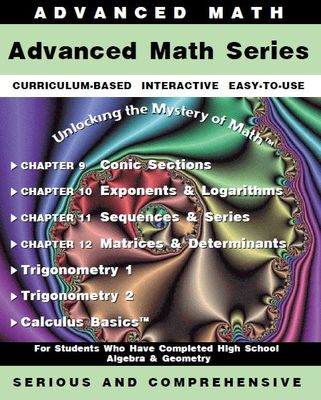Tutorial math and reading software for elementary and secondary arithmetic, basic math, algebra, geometry, precalculus plus GED, ABE, and CLEP preparation for elementary school, high school, college, adult education, and homeschool students.

# Advanced Math programs forAlgebra 2TrigonometryPre-CalculusCalculus BasicsPurchase Set of 7 Advanced Math programs
Regular price: \$349.00
Limited Time Offer: \$174.50
Media type::

## Product Description

The Advanced Math Software Series is educational software for continuing Advanced Algebra (Algebra 2),College Algebra, Trigonometry, and Pre-Calc students. This pre-calculus academic software series also provides math help for post high school algebra students. Graphing calculator lessons are scattered throughout the 7-program pre-calculus software series.

The first four educational software programs in this pre-calculus math software series are a continuation of the Algebra by Chapter series appropriate for high school math students as well as adult students. The Conic Section program provides in-depth practice to understanding, calculating, and graphing conic sections -- math help is always a click away. The Exponent and Logarithm program teach beginning through advanced exponents with log functions calculated both by hand and by calculator. Sequences and Series educational math software program provides interactive practice with formulas leading to the more advanced topics of binomial expansions. Matrices and Determinants concludes this 4-program high school math educational software series. Each of these four pre-calculus software programs has a word problem section complete with math help and concludes with a comprehensive test of the material learned in this math software program.

This academic software package contains Trigonometry 1 and Trigonometry 2 which together form a complete trigonometry software course and can be used to prepare for the CLEP exam or prepare for college placement exams. Concentrated learning of trigonometric functions and trigonometric identities provides the student with ample practice to form a solid understanding of Trig. Each of these two Trigonometry software programs concludes with a 30-question test with hints and complete solutions accessed upon student request.

The Calculus Basics educational software program contains a comprehensive pre-calculus software section to benefit college algebra students. The program then teaches the basics of first semester calculus (derivatives).
This 7-program academic software package includes the following pre-calculus and college algebra software titles:

"Conic Sections"
"Exponents and Logarithms"
"Sequences and Series"
"Matrices and Determinants"
"Trigonometry (Part 1)"
"Trigonometry (Part 2)"
"Calculus Basics" (with College Algebra review)

Available as: Downloadable for Windows, CD for Windows, CD for Macintosh, Online Subscription for Windows